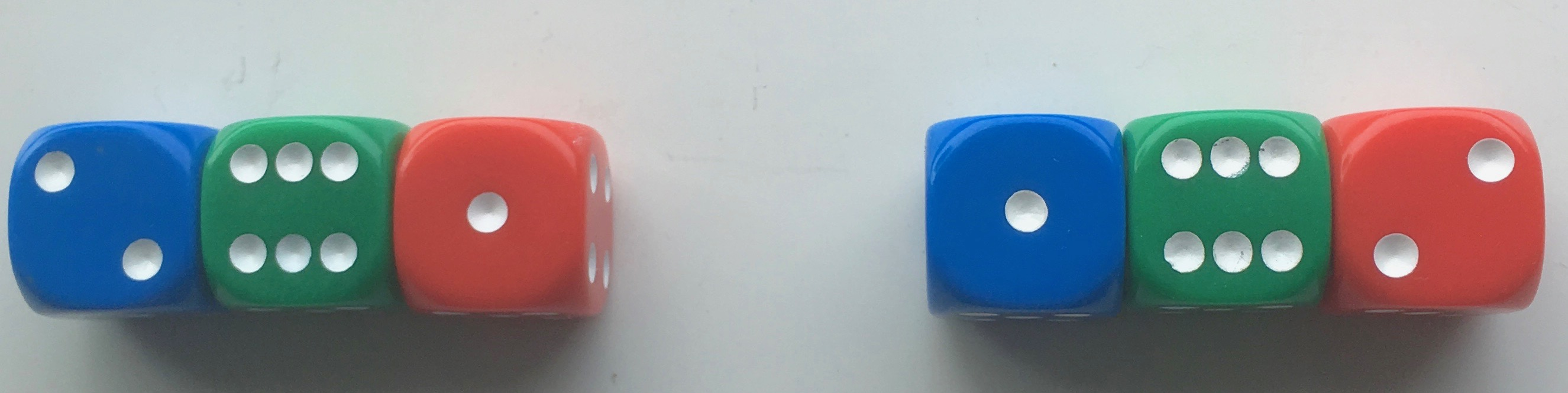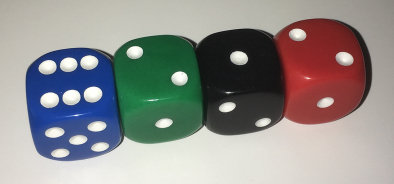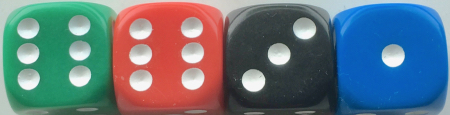#### You may also like### Prompt Cards

These two group activities use mathematical reasoning - one is numerical, one geometric.### Consecutive Numbers

An investigation involving adding and subtracting sets of consecutive numbers. Lots to find out, lots to explore.### Exploring Wild & Wonderful Number Patterns

EWWNP means Exploring Wild and Wonderful Number Patterns Created by Yourself! Investigate what happens if we create number patterns using some simple rules.

# Sticky Dice

##### Age 7 to 11Challenge Level

Throughout these challenges, the touching faces of any adjacent dice must have the same number.In the picture above, three dice are joined with 4s between the blue and green, and therefore 3s between the green and red.
The total of the numbers on the top is 9 in each case.
The two arrangements will be thought of as the same even though they are in a different order and colour. This is true for all the challenges.

For the challenges below, you'll be using four dice.

#### Challenge 1

Find a way of making the total on the top come to each number from 11 to 18 inclusive, keeping to the same joining numbers throughout.

Here is an example:Remember, any touching faces must be a pair of the same numbers.

#### Challenge 2

Using any joining numbers, find as many different ways as possible to make the tops add up to 12.
How can you be sure you've found all the possible ways?

#### Challenge 3

Arrange the dice in as many ways as possible, so the total of the tops of the left-hand pair is twice the total of the tops of the right-hand pair.

In the picture below, the top total of the left-hand pair is three times the right-hand pair, not two times so you cannot use this arrangement.#### Challenge 4

Arrange the dice in as many ways as possible, so the pair on the left-hand side has a top product* which is double or treble the right-hand pair product?

In the picture above, the left-hand pair product is twelve times bigger than the right.

*The product of two numbers is one multiplied by the other. For example, the product of 3 and 5 is 15 because 3x5=15.

Here is a sheet of the activity.